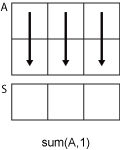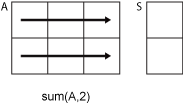# hdl.treeprod

Product of array elements using tree architecture

## Syntax

``B = hdl.treeprod(A)``
``B = hdl.treeprod(A, 'all')``
``B = hdl.treeprod(A, dim)``

## Description

example

````B = hdl.treeprod(A)` returns the product of the elements of A. If `A` is a vector, then `hdl.treeprod(A)` returns the product of the elements.If `A` is a matrix, then `hdl.treeprod(A)` returns a row vector containing the products of each column.The function `hdl.treeprod` uses a tree architecture to multiply elements. The tree architecture multiplication yields a shorter critical path, which leads to reduced latency when generating HDL code from a MATLAB Function block. When generating HDL code, the function `hdl.treeprod` reduces the amount of matching delays required to multiply elements.```

example

````B = hdl.treeprod(A, 'all')` computes the product of all elements of `A`.```

example

````B = hdl.treeprod(A, dim)` returns the product along dimension `dim`. For example, if `A` is a matrix, `hdl.treeprod(A,2)` is a column vector containing the products of each row.```

## Examples

### Product of Vector Elements

Create a vector and compute the product of its elements.

```A = 1:5; B = hdl.treeprod(A)```
```B = 120```

### Product of Matrix Elements

Create a matrix and compute the product of its elements.

`A = [1 3 2; 4 2 5; 6 1 4]`
```A = 3×3 1 3 2 4 2 5 6 1 4 ```
`B = hdl.treeprod(A,'all')`
```B = 5760 ```

### Product of Matrix Columns

Create a matrix and compute the product of the elements in each column.

`A = [1 3 2; 4 2 5; 6 1 4]`
```A = 3×3 1 3 2 4 2 5 6 1 4 ```
`B = hdl.treeprod(A)`
```B = 1×3 24 6 40 ```

### Product of Matrix Rows

Create a matrix and compute the product of the elements in each row.

`A = [1 3 2; 4 2 5; 6 1 4]`
```A = 3×3 1 3 2 4 2 5 6 1 4 ```
`B = hdl.treeprod(A,2)`
```B = 3×1 6 40 24 ```

## Input Arguments

collapse all

Input array, specified as a scalar, vector, or 2-D matrix.

Data Types: `single` | `double` | `int8` | `int16` | `int32` | `int64` | `uint8` | `uint16` | `uint32` | `uint64` | `fi`
Complex Number Support: Yes

Dimension to operate along, specified as a positive integer scalar. If no value is specified, then the default is the first array dimension whose size does not equal 1.

Dimension `dim` indicates the dimension whose length reduces to 1. The `size(S,dim)` is `1`, while the sizes of the other dimensions remain the same.

Consider a two-dimensional input array, `A`:

• `sum(A,1)` operates on successive elements in the columns of `A` and returns a row vector of the sums of each column.• `sum(A,2)` operates on successive elements in the rows of `A` and returns a column vector of the sums of each row.Data Types: `double` | `single` | `int8` | `int16` | `int32` | `int64` | `uint8` | `uint16` | `uint32` | `uint64`

## Output Arguments

collapse all

Product array, returned as a scalar, vector, or matrix.

The data type of `B` is the same data type as that of input `A`.

## Version History

Introduced in R2022a